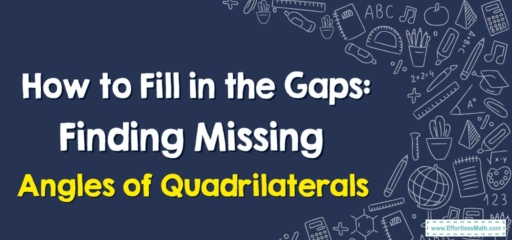# How to Fill in the Gaps: Finding Missing Angles of Quadrilaterals

Greetings, math detectives!
Today, we're turning our attention to geometry, specifically quadrilaterals. A quadrilateral is a polygon with four sides, and just like any other polygon, the sum of its interior angles can tell us a lot about its shape.A quadrilateral, as the name suggests, is a four-sided figure. Some common types of quadrilaterals include squares, rectangles, parallelograms, trapezoids, and rhombuses.

One key feature of quadrilaterals is that the sum of their interior angles always equals $$360$$ degrees.

## 2. Finding Missing Angles

Finding a missing angle in a quadrilateral involves some simple arithmetic once you know the sum of the interior angles.

## Step-By-Step Guide to Finding Missing Angles of Quadrilaterals

Let’s break down the process:

### Step 1: Add the Known Angles

First, add up the measures of the angles you know.

### Step 2: Subtract the Sum from 360

Next, subtract the sum of the known angles from $$360$$ degrees.

### Step 3: Find the Missing Angle

The result is the measure of the missing angle.

For instance, let’s say you’re given a quadrilateral with three known angles: $$90$$ degrees, $$110$$ degrees, and $$100$$ degrees:

1. Add the known angles: $$90 + 110 + 100 = 300$$ degrees.
2. Subtract this sum from $$360: 360 – 300 = 60$$ degrees.
3. So, the missing angle is $$60$$ degrees.

The art of finding missing angles is all about using the properties of the shape in question and a little bit of arithmetic.

Keep up the practice, and soon enough, you’ll be able to find missing angles in any polygon with ease. As always, keep exploring, keep practicing, and enjoy your mathematical journey!

In this blog post, we’ve explained how to find missing angles in quadrilaterals and provided a step-by-step guide. With a bit of practice, you’ll master this essential skill in no time. Happy angle hunting!

### What people say about "How to Fill in the Gaps: Finding Missing Angles of Quadrilaterals - Effortless Math: We Help Students Learn to LOVE Mathematics"?

No one replied yet.

X
30% OFF

Limited time only!

Save Over 30%

SAVE $5 It was$16.99 now it is \$11.99